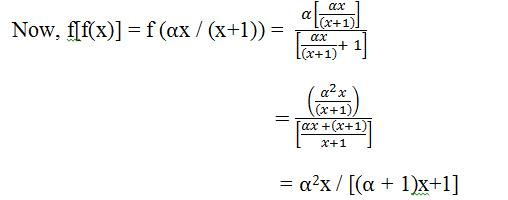Courses

# Notes | EduRev

## JEE : Notes | EduRev

The document Notes | EduRev is a part of the JEE Course JEE Main Mock Test Series 2020 & Previous Year Papers.
All you need of JEE at this link: JEE

Illustration 1: Let f(x) = αx / (x+1), x ≠ -1. Then, for what value of α is f(f(x) = x? (2001)
Solution: The given function f(x) = αx / (x+1), x ≠ -1Illustration 2: Suppose, f(x) = (x+1)2 for x ≥ -1. If g(x) is the function whose graph is a reflection of the graph of f(x) with respect to the line y = x, then what is the value of g (x)? (2002)
Solution: The question just requires us to find the inverse.
Let us assume y = f(x) = (x+1)2 for x ≥ -1
Then ± √y = (x+1) for x ≥ -1
Hence, √y = x + 1 which gives y ≥ 0 and x+1 ≥ 0.
Hence, x = √y - 1
So, f -1 (y) = √y - 1
Hence, f -1 (x) = √x – 1, x ≥ 0.

Illustration 3: Let f(x) = x2 and g(x) = sin x for all x ∈ R. Then, the set of all x satisfying (fogogof)(x) = (gogof)(x), where (fog)(x) = f(g(x)), is                                    (2011)
1. ±√nπ, n ∈ {0, 1, 2, ….}
2. ±√nπ, n ∈ {1, 2, …. }
3. π/2 + 2nπ, n ∈ {….. -2, -1, 0, 1, 2, ….}
4. 2nπ, n ∈ {….. -2, -1, 0, 1, 2, ….}
Solution: Let f(x) = x2 and g(x) = sin x
Then (gof) (x) = sin x2
Hence, go (gof) (x) = sin (sin x2)
Therefore, (fogogof) (x) = (sin (sin x2))2
Again, (gof) (x) = sin x2
So, (gogof) (x) = sin (sin x2)
Given, (fogogof) (x) = (gogof) (x)
Also, (sin (sin x2))2 = sin (sin x2)
Hence, we get, sin (sin x2).{sin (sin x2) – 1} = 0
This gives either sin (sin x2) = 0 or sin (sin x2) = 1
Hence, sin x2 = 0 or sin x2 = π /2
Therefore, x2 = nπ (but this is not possible since -1 ≤ sin θ ≤ 1.
So, x = ± √ nπ.

Offer running on EduRev: Apply code STAYHOME200 to get INR 200 off on our premium plan EduRev Infinity!

,

,

,

,

,

,

,

,

,

,

,

,

,

,

,

,

,

,

,

,

,

;